Function Repository Resource:

# WorldTravellerSignpostSolve

Find the location of a signpost with given distances to a set of cities

Contributed by: Sander Huisman
 ResourceFunction["WorldTravellerSignpostSolve"][{{loc1, dist1},{loc2, dist2},…}] given the locations loci and distances disti find the location of the signpost.

## Details

The algorithm finds the location p for which the sum of distance errors (distance from p to loci minus disti) squared is minimized: ∑i=1n(||p-loci||-disti)2 All locations loci are included in the calculation. The error between the distance from p to loci and disti can be anything such that even crude values will give a satisfactory answer. In general the crudeness/precision of the distances given gives the precision of the found location.

## Examples

### Basic Examples (1)

Given a set of cities and their distance, what is the location of the signpost:

 In:=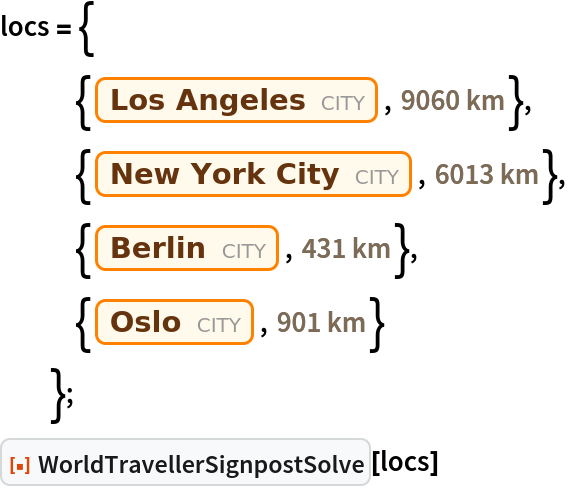Out=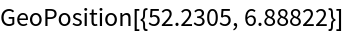### Scope (1)

Numbers are interpreted as kilometers:

 In:=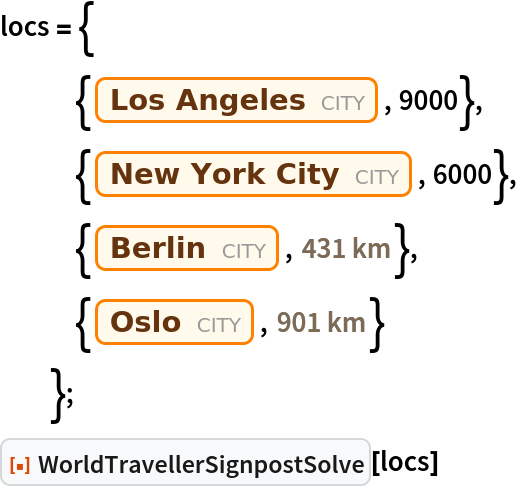Out=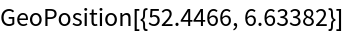### Neat Examples (2)

Given the signpost, find the location: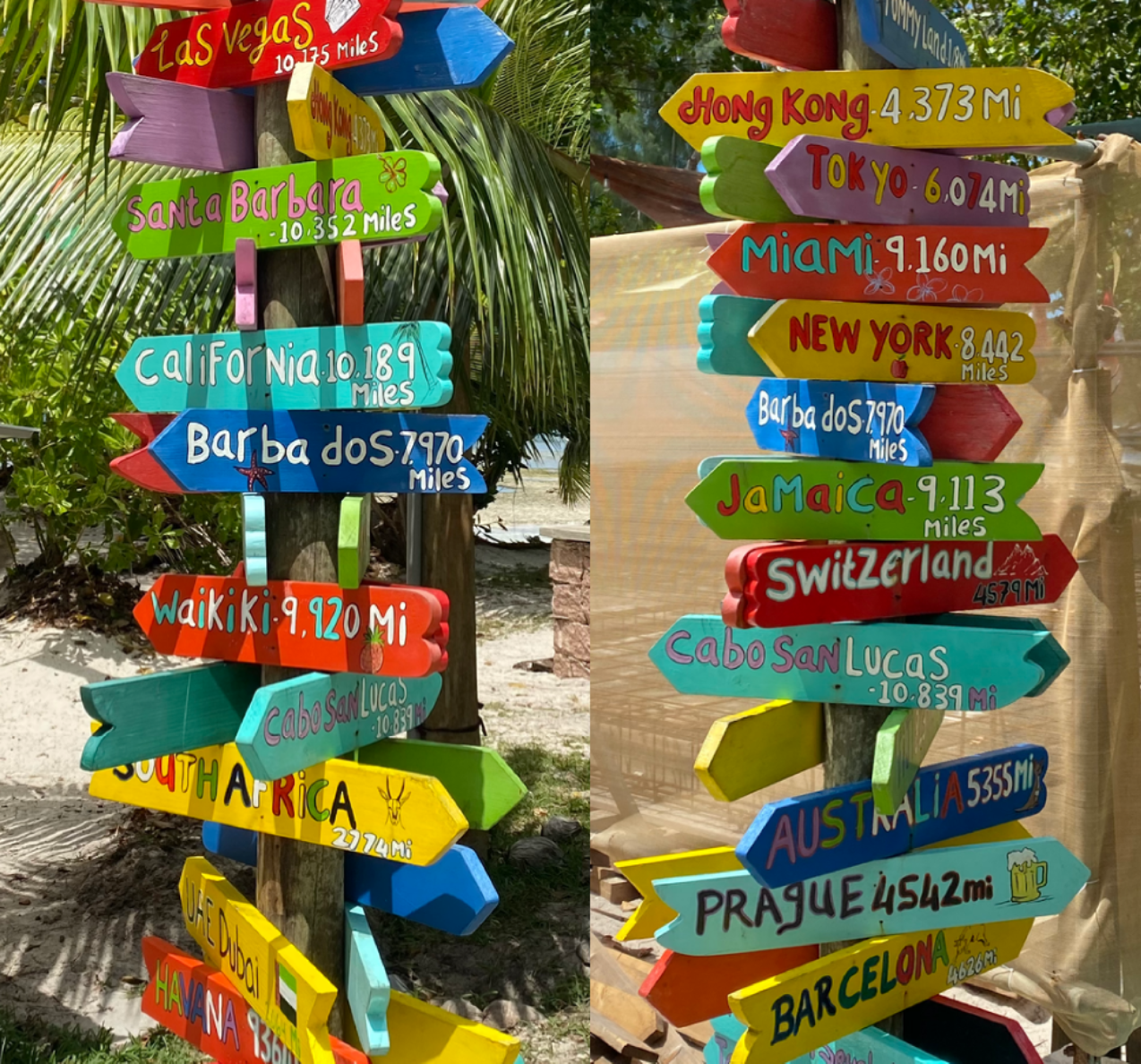In:=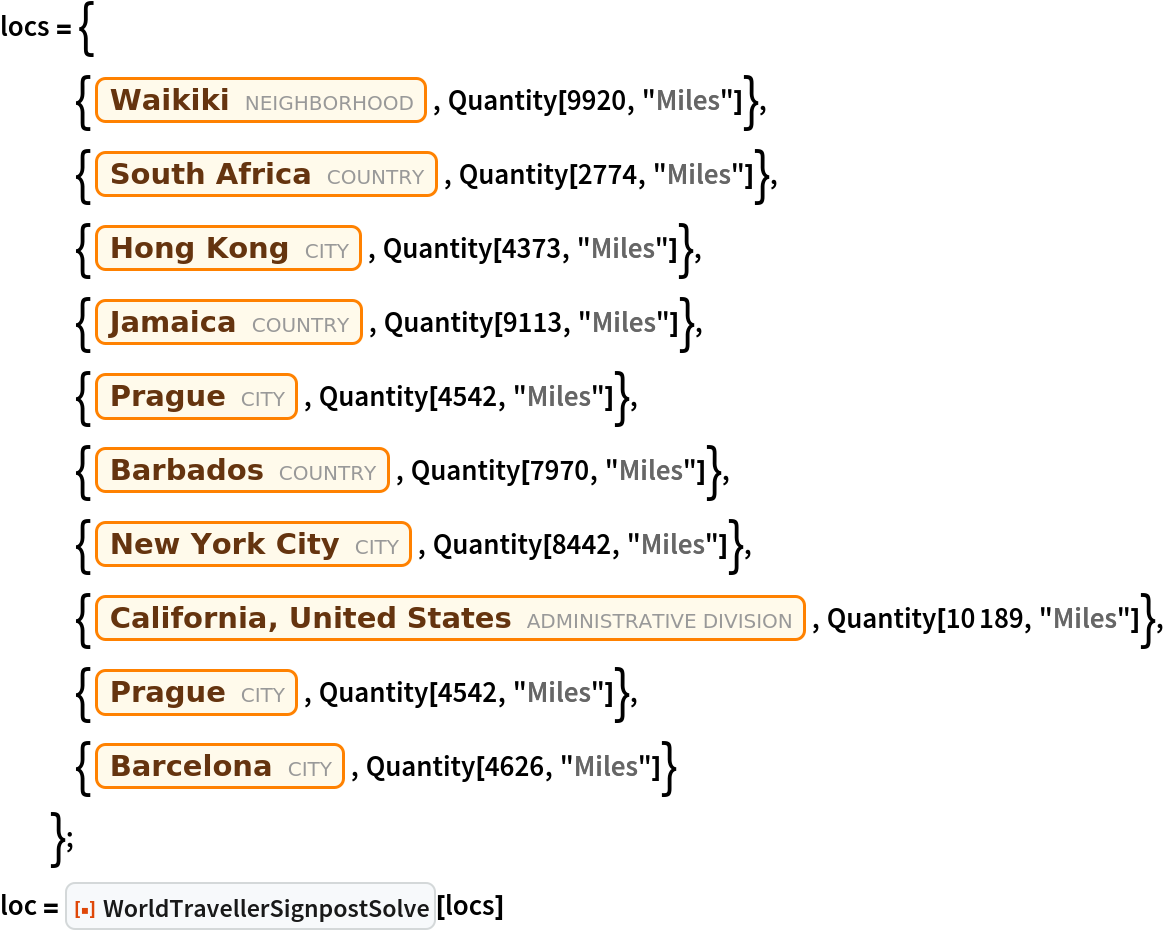Out=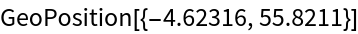Find the country:

 In:=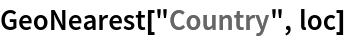Out=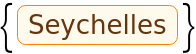Visualize the lines and the found minimum:

 In:=Out=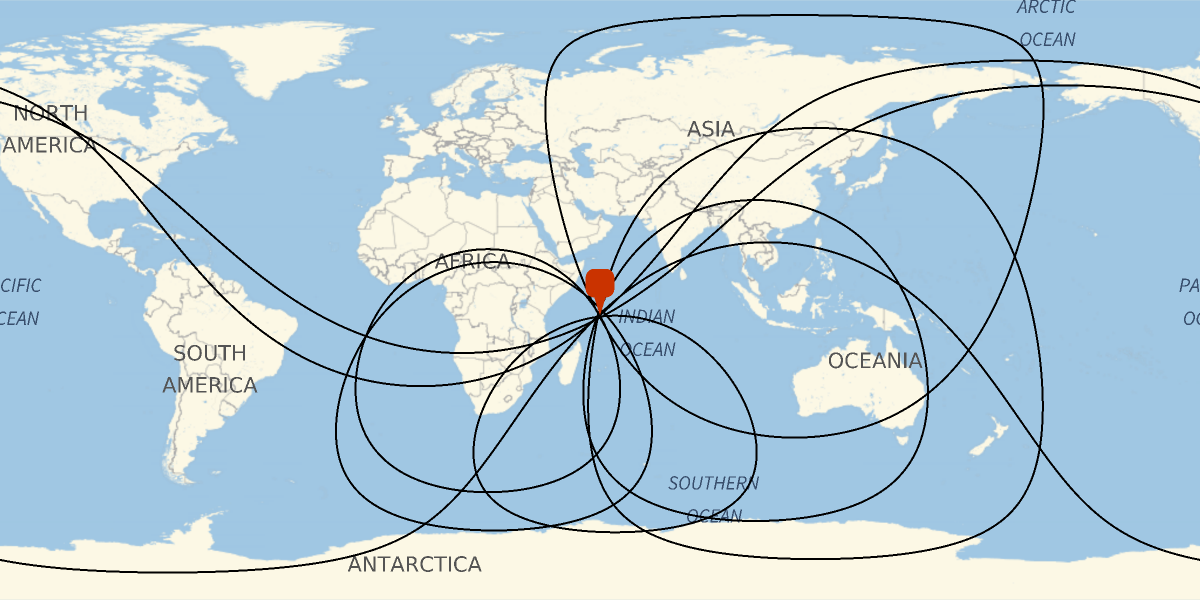Investigate the effect of the precision of the distances on the final location. Given a location, and some random cities, find the estimates for 'rounded' distances:

 In:=Out=Plot the error in the location as a function of the rounding:

 In:=Out=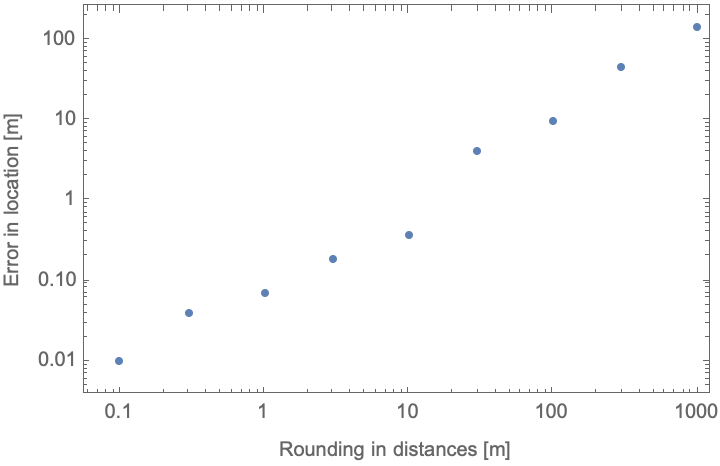We see that the error in the location is much better than the errors introduced by rounding.

SHuisman

## Version History

• 1.0.0 – 19 May 2023